Function Repository Resource:

# KhatriRaoProduct

Evaluate the Khatri-Rao product of matrices

Contributed by: Jan Mangaldan
 ResourceFunction["KhatriRaoProduct"][m1,m2,…] constructs the Khatri-Rao product of the matrices mi.

## Details

The Khatri-Rao product is a columnwise Kronecker product of matrices; that is, each column of the Khatri-Rao product is the Kronecker product of the corresponding columns of the mi.
The matrices mi must all have the same number of columns.

## Examples

### Basic Examples (1)

Khatri-Rao product of two matrices:

 In:=In:=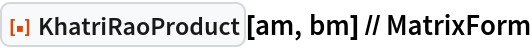Out=### Scope (2)

a and b are matrices with exact entries:

 In:=Use exact arithmetic to compute the Khatri-Rao product:

 In:=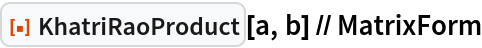Out=Use machine arithmetic:

 In:=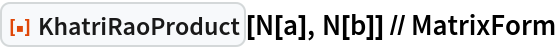Out=Use 20-digit precision arithmetic:

 In:=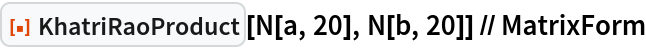Out=Evaluate the Khatri-Rao product of three matrices:

 In:=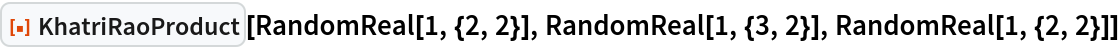Out=### Properties and Relations (3)

The Khatri-Rao product is multi-linear (linear in each argument):

 In:=In:=Out=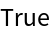In:=Out=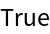The Khatri-Rao product is associative:

 In:=Out=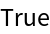The Khatri-Rao product is not commutative:

 In:=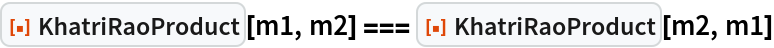Out=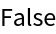## Version History

• 1.0.0 – 11 April 2022